# Extrapolatory Analysis — Does a “More Democratic” Society lead to an improvement in the “quality of life”?

As part of the Data Management and Visualisation course on Coursera, I am investigating the following hypothesis from the Gapminder dataset

What effect does the “level of democracy” in a country have on the “quality of life” of the country’s citizens?

I carried out a brief extrapolatory analysis of the dataset to figure out the distribution of the main variables that I shall be using to test my hypothesis. In short, I would be measuring the correlation between the “polityscore” (a numeric value from -10 to 10 that measures how democratic a country is)variable and the following variables in the dataset

• incomeperperson(2010 GDP per capita in constant US\$ 2000)

• alconconsumption(2008 alcohol consumption per adult)

• breastcancerper100TH(breast cancer new cases per 10,000)

• co2emissions(2006 cumulative co2 emissions)

• femaleemployrate(2006 female employment rate)

• employrate(2007 total emloyees over 15+)

• HIVrate(2009 estimated HIV prevalence)

• lifeexpectancy(2011 life expectancy at birth)

• suicideper100TH(suicide rate adjusted over 10,000 people)

• urbanrate(Urban population)

I carried out my analysis in Python, using the numpy and the pandas libraries. The entire script can be accessed from here.

The following is a run down of the script, along with an explanation of the insights that I gleamed from it.

``````import pandas as pd
import numpy as np
data = pd.read_csv(‘gapminder.csv’, low_memory=False)
print “Number of observations:”
print (len(data))
print “Number of variables:”
print (len(data.columns))
``````

The code snippet above displays the following:

The total number of rows in the dataset are 213, while the total number of variables (columns) are 16.

The next step was to convert all the variables that would be used in the analysis to numeric values. The following code snippet was used for this

``````#setting variables you will be working with to numeric
data[‘incomeperperson’] = data[‘incomeperperson’].convert_objects(convert_numeric=True)
data[‘alcconsumption’] = data[‘alcconsumption’].convert_objects(convert_numeric=True)
data[‘breastcancerper100th’] = data[‘breastcancerper100th’].convert_objects(convert_numeric=True)
data[‘co2emissions’] = data[‘co2emissions’].convert_objects(convert_numeric=True)
data[‘femaleemployrate’] = data[‘femaleemployrate’].convert_objects(convert_numeric=True)
data[‘employrate’] = data[‘employrate’].convert_objects(convert_numeric=True)
data[‘hivrate’] = data[‘hivrate’].convert_objects(convert_numeric=True)
data[‘lifeexpectancy’] = data[‘lifeexpectancy’].convert_objects(convert_numeric=True)
data[‘suicideper100th’] = data[‘suicideper100th’].convert_objects(convert_numeric=True)
data[‘urbanrate’] = data[‘urbanrate’].convert_objects(convert_numeric=True)
data[‘polityscore’] = data[‘polityscore’].convert_objects(convert_numeric=True)
``````

I also wanted to ensure that only those values were being considered in the analysis which had no missing values for any of the variables that were to be considered. To achieve this, I used the following code snippet:

``````#select data subset that does not have any missing polity scores
subset = data[np.isfinite(data[‘polityscore’])]
subset = subset[np.isfinite(subset[‘incomeperperson’])]
subset = subset[np.isfinite(subset[‘alcconsumption’])]
subset = subset[np.isfinite(subset[‘breastcancerper100th’])]
subset = subset[np.isfinite(subset[‘co2emissions’])]
subset = subset[np.isfinite(subset[‘femaleemployrate’])]
subset = subset[np.isfinite(subset[‘employrate’])]
subset = subset[np.isfinite(subset[‘hivrate’])]
subset = subset[np.isfinite(subset[‘lifeexpectancy’])]
subset = subset[np.isfinite(subset[‘suicideper100th’])]
subset = subset[np.isfinite(subset[‘urbanrate’])]
``````

A frequency table for the polityscore variable was achieved with the following

``````group = subset.groupby(‘polityscore’).size()
print group
``````

The display was as follows

However, I decided against creating frequency tables for the other variables that I shall be using in my analysis, since most of them are continuous variables and by merely plotting the frequency tables, no worthwhile analysis could be inferred.

Instead, I decided to plot boxplots for all of the variables in consideration against the polityscores. This would give a good picture of how exactly each variable changed with how “democratic” or “autocratic” a country was.

The following code was used to draw the different boxplots.

``````subset.boxplot(column=’incomeperperson’,by=’polityscore’)
subset.boxplot(column=’alcconsumption’,by=’polityscore’)
subset.boxplot(column=’breastcancerper100th’,by=’polityscore’)
subset.boxplot(column=’co2emissions’,by=’polityscore’)
subset.boxplot(column=’femaleemployrate’,by=’polityscore’)
subset.boxplot(column=’employrate’,by=’polityscore’)
subset.boxplot(column=’hivrate’,by=’polityscore’)
subset.boxplot(column=’lifeexpectancy’,by=’polityscore’)
subset.boxplot(column=’suicideper100th’,by=’polityscore’)
subset.boxplot(column=’urbanrate’,by=’polityscore’)
``````

The following are the boxplots that were generated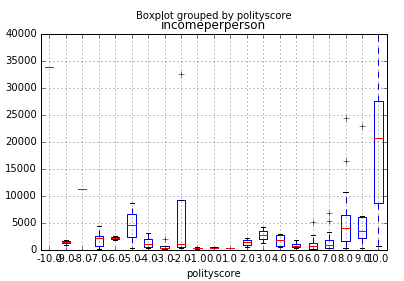Boxplot of Average Income per person against polityscore

There is a strong positive correlation between how “democratic” an economy is and the average annual income level.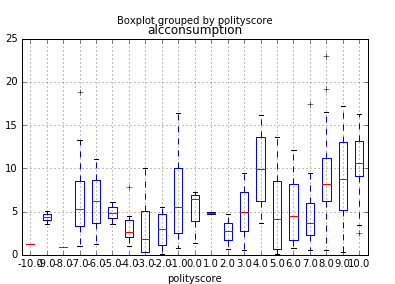Boxplot of Alcohol consumption against polityscore

Surprisingly, the amount of alcohol consumption goes up as an economy becomes more ‘democratic’. However, this increase might only be due to a country becoming more “open”. There is not much data to infer whether this increased alcohol consumption actually leads to any detrimental effects in the economy.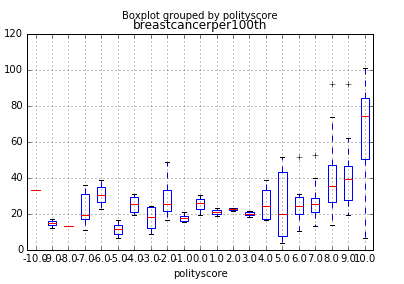Boxplot of Rates of Breast Cancer cases per 1000 people against polityscore

The rates of breast cancer rise up as the economy becomes more “open”. This is a surprising result, since one would believe that the general level of health and the prevalence of diseases might go down as a country becomes more democratic. However, a possible explanation of this trend might be that a more ‘open’ society leads to more cases of breast cancer being reported, since better healthcare leads to more women being tested for it.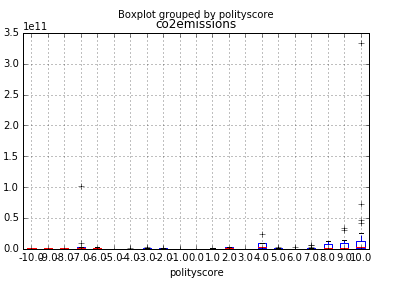Boxplot of CO2 emission against polityscore

There is a very slight positive correlation between the polityscore and the levels of CO2 emissions in a country. A possible reason of this might be that increased industrialisation in more “open” societies leads to more level of co2 emissions.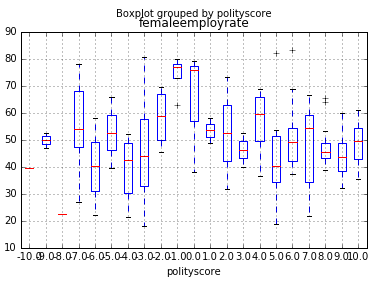Boxplot of female employment against polityscore

A correlation cannot be drawn explicitly between the level of “democracy” in a society and the level of female employment. While proponents of democracy argue that democracy and women’s rights go hand-in-hand, it would be wrong to equate the level of female employment in a country to be the only metric for measuring how well the women are generally treated in it. For instance, a reason for the high level of female employment in more ‘autocratic’ countries might be that women often have to work to support their families.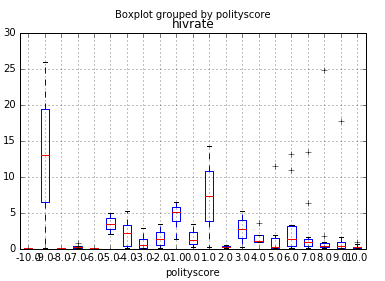Boxplot of HIV rates against polityscore

There is a strong negative correlation between the rates of HIV in a country and how “democratic” it is, which points to an improvement in the healthcare of a country as it becomes more ‘democratic’.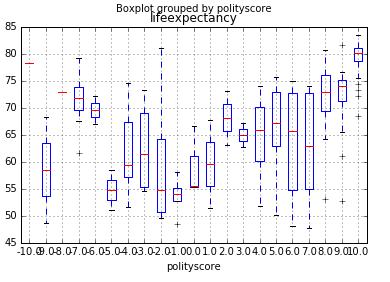Boxplot of life expectancy against polityscore

There is a positive correlation between the life expectancy of a country and its level of ‘democracy’.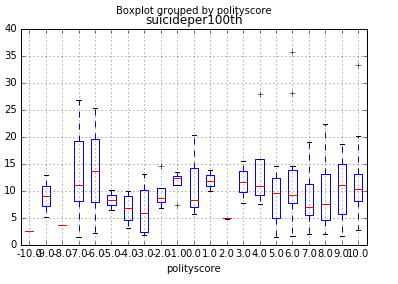Boxplot of suicide rate per 1000 against polityscore

Much cannot be deduced about the suicide rate of a country being linked with the level of ‘democracy’ in a country. The rate fluctuates across different democracies, which hints at the fact that there must be other reasons that are related more strongly to the suicide rate of a country.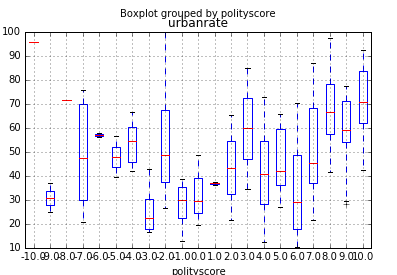Boxplot of urban rate against polityscore

The rate of urbanisation in a country is strongly positively correlated with how “democratic” it is.

Based on the analysis, I shall now be considering the following variables in my analysis:

• alconconsumption(2008 alcohol consumption per adult)

• breastcancerper100TH(breast cancer new cases per 10,000)

• employrate(2007 total emloyees over 15+)

• HIVrate(2009 estimated HIV prevalence)

• lifeexpectancy(2011 life expectancy at birth)

• urbanrate(Urban population)

This post was originally published on Medium